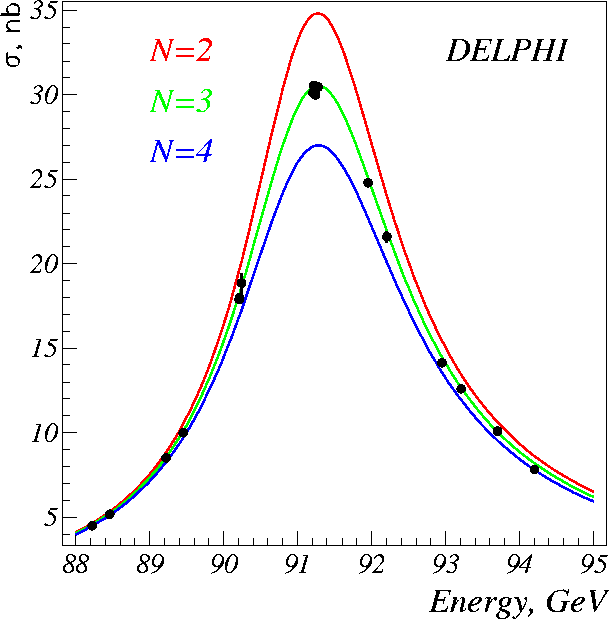The Z Particle Branching Ratios and the Number of Families

The official values of the Z branching ratios, taken by combining the results from all four CERN experiments are as follows:
• Branching ratio to electron-positron pairs: (3.366+/- 0.008)%
• Branching ratio to muon-antimuon pairs: (3.367+/- 0.013)%
• Branching ratio to tau-antitau pairs: (3.360+/- 0.015)%
• Branching ratio to quark-antiquark pairs: (69.90+/- 0.15)%
Do your numbers agree? If not, can you guess why?

The reason is the particles called neutrinos that escape the detector unseen. These account for about 20% of all Z particle decays, that's why if you add up the branching ratios to visible particles (listed above), you only get 80%.

You can correct your results for invisible decays quickly by dividing the total number of events you looked at by 0.8 and then recalculating your branching ratios with this as the total number of events. So, for example, if you looked at all 100 events, you would get 125. In other words, you are assuming that for those 100 events, 25 Zs were produced and decayed into neutrinos unseen by the detector, and are not in the file.

Do your recalculated results agree with the published ones?

What does all this mean?

Measuring branching ratios is a way of finding out about how particle interactions work in detail. First of all, notice how similar the branching ratios to electron-positron pairs, muon-antimuon pairs and tau-antitau pairs are. That is because of a phenomenon called lepton universality. Recall the Standard Model of particles:If you have read the pages about matter and interactions, you'll remember that matter particles are grouped into three families. The lightest family consists of particles called up-quarks, down-quarks, electrons and electron-neutrinos. It is the quarks and electrons from this family that make up all of ordinary matter.

The heavier families are made up of particles very similar to but more massive than those of the lightest family. The muons and taus are the heavy cousins of the electrons, and they each have associated neutrinos. Collectively, the electrons, muons, taus and neutrinos are called leptons.

As long as the energy of a collision is high enough to make the heavier leptons, nature shows no preference for one kind of charged lepton over any other. That's what lepton universality means, and it's why the branching ratio is the same for electron-positron pairs, muon-antimuon pairs and tau-antitau pairs.

Now think about the electrically neutral leptons, the neutrinos. Nature also treats all of them equally, so a Z is just as likely to decay into a neutrino-antineutrino pair of the electron, muon, or tau type.

But there is a difference between the neutral and charged leptons. It turns out that a Z is twice as likely to decay into a neutral lepton-antilepton pair as into a charged lepton-antilepton pair. That's why the Z decays we see in the detector account for only about 80% of the total.

The Number of Particle Families

The branching ratios of the Z particle give us a way of working out just how many families of particles there are in the Universe. For the purposes of this analysis, we've assumed there are three families and used that assumption to make your results agree with the published ones. What was done in the real LEP experiments, however, is that the probability of a Z decaying to produce particles visible to the detector was measured as a function of collision energy. The result is a graph that looks like this:The three coloured lines correspond to what the theory predicts the graph should look like for two, three and four families of particles. Notice that the peak is highest for two families and lowest for four. That's because the smaller the number of families, the greater the fraction of Z decays that produces visible particles, and the higher the peak. The data fit very well with the three-families line, which tells us that if our theories are right, then the three families of particles we already know about are all there is. What it doesn't tell us, however, is why nature has chosen to make two copies of the basic family of particles. That is a question for another generation of physicists to address. Maybe you?